# Stage 3

## Overview

In the third stage of the Cityscape Challenge, we add variety to the buildings by varying the types of windows in the offices and the types of roofs on the buildings. Then, we create and draw a random building.

## Draw Buildings with Four Types of Windows

In this example, we modify the `drawFrenchFlag()` function to draw the flags of France, Colombia, and Thailand.

We tell the `drawFlag()` function which flag to draw by passing it a value that is stored in the `country` parameter. Then, in the switch statement, we draw a French flag if `country == 'France'`, a Colombian flag if `country == 'Colombia'`, and a Thai flag if `country == 'Thailand'`.

Try drawing a Thai flag instead of a Colombian flag to see what it looks like. To learn more about switch statements, visit the Switch Statements lesson.

Quick Reference:

Message Log

## Challenge 1

Update the `drawBuilding()` function that you wrote in Stage 2 Challenge 3 to draw four types of windows.

Start by adding a `windowType` parameter to the `drawBuilding()` function:

``````function drawBuilding(x, y, units, floors, windowType) {

// code block

}``````

Inside the `drawBuilding()` function, remove the code you wrote to draw a rectangle for each window, and replace it with:

``drawWindow(windowType);``

This passes the value passed into the `drawBuilding()` function's `windowType` parameter into the `drawWindow()` function's `windowType` parameter. Both parameters happen to share the same name, but that isn't necessary.

Since all four window types use the same dark gray color (`'#666666'`), set the `context.fillStyle` property in the `drawBuilding()` function before calling the `drawWindow()` function.

Then, find the definition for the `drawWindow()` function in the program below:

``````function drawWindow(windowType) {

// YOUR CODE FOR DRAWING THE FOUR DIFFERENT TYPES OF WINDOWS HERE

}``````

Inside the `drawWindow()` function, add a switch statement with four cases. We will draw four different types of windows depending if `windowType` is 0, 1, 2, or 3.

The dimensions of the four window types are given below: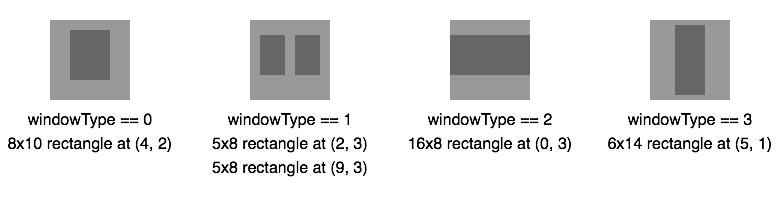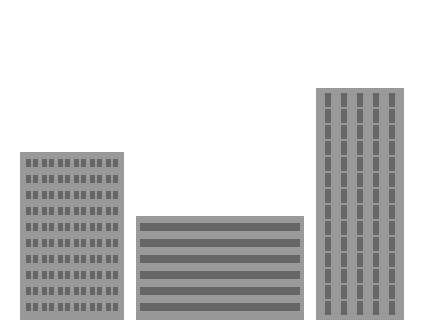What your drawing should look like

Draw three buildings with different sizes and window types.

The first building sits on the ground at (20, 320) with 6 office units per floor, 10 floors, and type 1 windows.

The second building sits on the ground at (136, 320) with 10 office units per floor, 6 floors, and type 2 windows.

The third building sits on the ground at (316, 320) with 5 office units per floor, 14 floors, and type 3 windows.

Quick Reference:

Previous Challenge: View your code from Stage 2 Challenge 3 to use on this challenge.

Code Missing: You have not yet entered any code in to the previous challenge: Stage 2 Challenge 3
Stage 2 Challenge 3
Message Log
##### Canvas (your drawing will display here)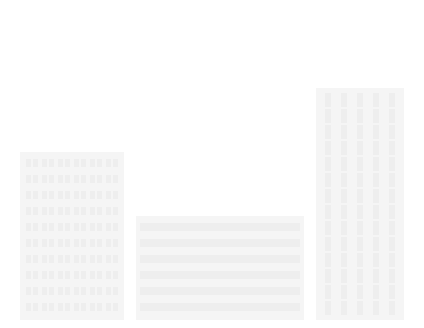## Draw Buildings with Four Types of Roofs

In this example, we add a triangle to the top of the building's roof.

To draw the roof, we create a function called `drawRoof()` and pass it the width of the building. We pass it the width of the building because we want to center the roof and scale the roof to fit the building.

``````function drawRoof(w) {

// code block

}``````

Inside the `drawRoof()` function, to make the roof a little easier to draw, we use the `context.translate()` method to move to the center of the roof and the top of the triangle.

``context.translate(w / 2, -96); // Move to the center of the roof and top of the triangle``

Then, we use the `context.lineTo()` method to create a triangular path and the `context.fill()` method to fill it. Because the roof is the same color as the building, we don't have to set the `context.fillStyle` property.

``````context.beginPath(); // Start a new path
context.moveTo(0, 0); // Move to the top of the triangle
context.lineTo(16, 64); // Create a line to the bottom right vertex of the triangle
context.lineTo(-16, 64); // Create a line to the bottom left vertex of the triangle
context.closePath(); // Close the triangle
context.fill(); // Fill the triangle with the current fill color``````

After creating and filling the triangle, we draw two rectangles beneath it. The width of the second rectangle varies depending on the width of the building.

``````context.fillRect(-24, 64, 48, 16);
context.fillRect(-(w - 16) / 2, 80, w - 16, 16);``````

Change the number of office units on a floor of the building to a number between 4 and 8. Note how the roof adjusts to fit the size of the building.

The roof doesn't work when the number of office units is smaller than 4 and it doesn't look good when it's greater than 8. We will fix those issues later.

To learn more about drawing triangles, visit the lineTo() lesson.

Quick Reference:

Message Log

## Challenge 2

Update the `drawBuilding()` function to draw four types of roofs.

Start by adding a `roofType` parameter to the `drawBuilding()` function definition. Then, inside the `drawBuilding()` function, draw the roof after drawing the building's gray rectangle and before translating the coordinate system or changing the `context.fillStyle` property to draw the windows.

``drawRoof(w, roofType);``

Inside the `drawRoof()` function, add a switch statement with four cases.

The dimensions of the four window types are given below, where `w` is the width of the building: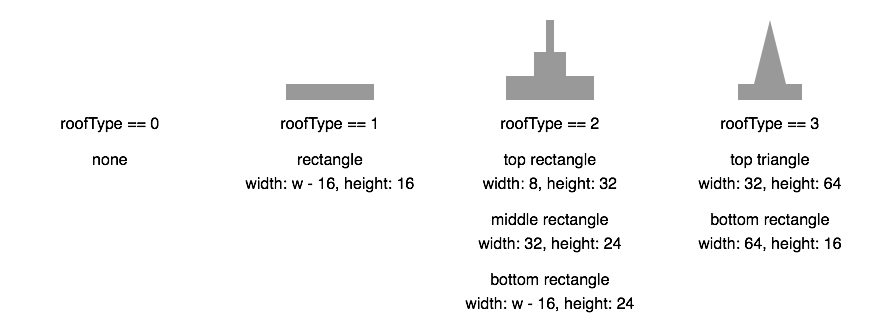Since all four roof types use the same gray color as the building (`'#999999'`), we don't have to change the `context.fillStyle` property in the `drawBuilding()` function before drawing the roof. Also, for a case that does nothing, either leave the case out or include the case with a comment explaining what it is supposed to do (make sure to include the break statement).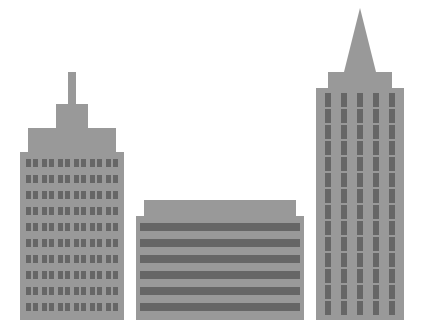What your drawing should look like

Draw three buildings with different sizes and window and roof types.

The first building sits on the ground at (20, 320) with 6 office units per floor, 10 floors, type 1 windows, and a type 2 roof.

The second building sits on the ground at (136, 320) with 10 office units per floor, 6 floors, type 2 windows, and a type 1 roof.

The third building sits on the ground at (316, 320) with 5 office units per floor, 14 floors, type 3 windows, and a type 3 roof.

Quick Reference:

Previous Challenge: View your code from Stage 3 Challenge 1 to use on this challenge.

Code Missing: You have not yet entered any code in to the previous challenge: Stage 3 Challenge 1
Stage 3 Challenge 1
Message Log
##### Canvas (your drawing will display here)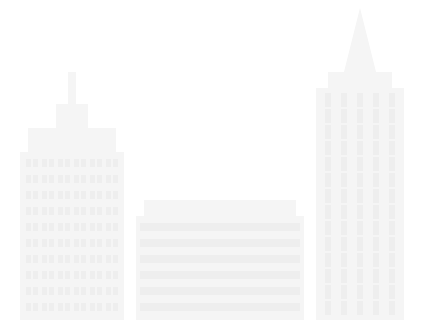## Draw Buildings with a Random Number of Floors

Right now, we have a `drawBuilding()` function that draws a building if we tell it how many office units per floor, how many floors, the type of windows, and the type of roof the building has. The next step is generating a building randomly, starting with the number of floors.

In this example, we draw a random number of flags and select the type of flag randomly.

We start by assigning a random integer between 0 and 2 to the `country` variable. We have modified the switch statement inside the `drawFlag()` function so `country == 0` is France, `country == 1` is Colombia, and `country == 2` is Thailand.

``var country = Math.floor(3 * Math.random()) // Generates a random integer between 0 and 2``

We generate a random number using the `Math.random()` function, which returns a random number greater than or equal to 0 and less than 1.

By multiplying `Math.random()` by 3, we get a random number between 0 and 3. However, the random number will be a decimal, something like 1.748. To convert it to an integer, we use the `Math.floor()` function to round the number down. This will give us a random integer between 0 and 2, so either 0, 1, or 2. The reason we will never get a 3 is because `3 * Math.random()` is always less than 3 and we are rounding down.

We are using a for loop to draw multiple flags. The number of flags it draws is a random integer between 2 and 5 assigned to the `flagCount` variable:

``var flagCount = 2 + Math.floor(4 * Math.random()); // Generates a random integer between 2 and 5``

To get a random integer between 2 and 5, we start by getting a random integer between 0 and 3. Since there are four integers between 0 and 3, we use `Math.floor(4 * Math.random())`. Note that we are multiplying `Math.random()` by 4, not 3. Then, to increase the random integer to between 2 and 5, we simply add 2.

Press "Run" to change the number and type of flag drawn. Can you figure out how you would edit the code to randomize the type of each individual flag in the drawing?

To learn more about rounding and generating random numbers, visit the round() / floor() / ceil() and random() lessons.

Quick Reference:

Message Log

## Challenge 3

Instead of passing a number into your `drawBuilding()` function for the number of office units per floor, pass it a variable that's been assigned a random integer between 6 and 20. Since there are 15 integers between 6 and 20, make sure to multiply `Math.random()` by 15, not 14.

Draw a building sitting on the ground at (50, 320) with 12 office units on a floor, a random number of floors, type 0 windows, and a type 1 roof.

Press "Run" multiple times to make sure the `drawBuilding()` function is drawing a building with a random number of floors between 6 and 20 Then, once you feel satisfied with your drawings, mark the challenge as complete by selecting "Yes, it looks good".

Quick Reference:

Previous Challenge: View your code from Stage 3 Challenge 2 to use on this challenge.

Code Missing: You have not yet entered any code in to the previous challenge: Stage 3 Challenge 2
Stage 3 Challenge 2
Message Log

## Draw Completely Random Buildings

Now that you know how to randomize the number of floors in a building, you are going to randomize the number of office units, the type of windows, and the type of roof, too.

But before you do that, we are going to create a function for generating random integers.

The `Math.random()` function is great for generating random decimals, but generating random integers is more complicated. It takes more steps and it's easy to make a mistake.

In this example, we create the `randomInteger()` function, which takes an integer assigned to the parameter `max` and returns a random integer between 0 and `max`. For example, `randomInteger(10)` returns a random integer between 0 and 10, including 0 and 10.

Because the number of integers between 0 and `max` is actually `max + 1`, we use `Math.floor((max + 1) * Math.random())` to generate the random integer and a return statement to return it.

We use the `randomInteger()` function to draw a rectangle with a random size and position. Press "Run" to see the rectangle's size and position change.

To learn more about returning values from functions, visit the Functions lesson.

Quick Reference:

Message Log

## Challenge 4

Before we can use the `randomInteger()` function to draw random buildings, we need to make a change to it.

Right now, `randomInteger()` returns an integer between 0 and `max`. Since we don't want to draw buildings with 0 floors or 0 office units per floor, we need it to return an integer between `min` and `max`, including `min` and `max`.

Change the `randomInteger()` function so it returns an integer between a `min` value and a `max` value, including those two values. Remember that to get a random integer between 0 and 14, we multiply `Math.random()` by 15, not 14, because there are 15 integers between 0 and 14.

Then, use the `randomInteger()` function to draw a random building sitting on the ground at (50, 320) with 4-10 office units per floor, 6-20 floors, and random window and roof types.

Note: If you want to restrict the type of roof depending on the number of office units per floor, we can do that with an if statement. While we aren't going to introduce if statements in this challenge, this is the code we used to generate a random roof type:

``````var roofType;

if (units > 8) {
roofType = randomInteger(0, 1);
} else if (units > 6) {
roofType = randomInteger(0, 2);
} else {
roofType = randomInteger(0, 3);
}``````

Because we are using the number of office units per floor, assigned to the variable `units`, make sure to generate a random value for `units` first.

Press "Run" multiple times to see if the `drawBuilding()` function is actually drawing a random building. Make sure you see buildings with type 3 windows and roofs. A building with no windows, means the program is generating a random `windowType` not covered by the cases in the `drawWindow()` function's switch statement. Once you feel satisfied with your drawings, mark the challenge as complete by selecting "Yes, it looks good".

Quick Reference:

Previous Challenge: View your code from Stage 3 Challenge 3 to use on this challenge.

Code Missing: You have not yet entered any code in to the previous challenge: Stage 3 Challenge 3
Stage 3 Challenge 3
Message Log

## Ready for the next lesson?

Next up, the "Stage: 4" lesson >